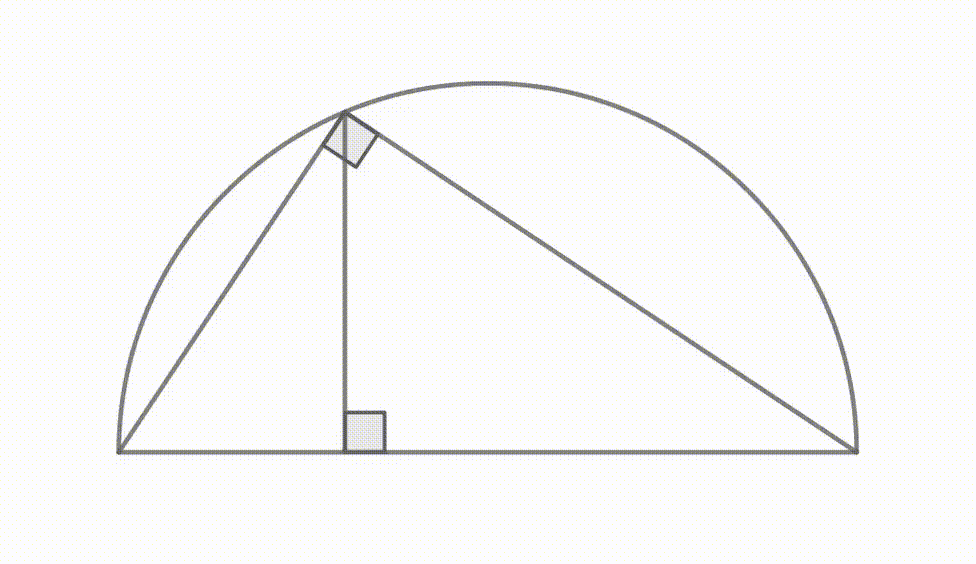Fun with triangles: Proving a simple theorem

Welcome.

Some context

I'm working with the book Famous Problems of Geometry and How to Solve them.

What is synthetic geometry?

It's just like the geometry you've heard of but with some constraints. Our only tools are a ruler (not to measure distances but to draw straight lines) and a compass, to draw curves.

Prerequisites

Well, you have to know what a right-angle is, stuff like that. Not a really hard post.

The problemBased on this picture, we should be able to tell BN's length in terms of AN and NC. In fact, we want to prove that BN² = AN · NC.

Do not mistake this relation with the Pythagorean theorem; which states that AC² = AB² + BC²!!

How to build this figure? (Optional)

Step 1 is to draw a straight line segment. We don't care about its length. Based on our figure, this segment is segment AC.

Step 2 is to draw a semi-circle (again, we don't care about its diameter). Simply, stick you compass somewhere on the segment you just drew and draw a semi-circle (or a full circle but we only care about half the circle, the one that goes from A to C).

Hint: place A and C after drawing the semi-circle.

Now using your compass and a ruler, draw another line segment. Make this one perpendicular to the first segment. It must at least touch the semi-circle. We'll name it BN.

Now, the last step is to draw the two other sides of the triangle. Simply by connecting the corners of our circle with the point where segment n°2 touches the semi-circle. You can do this with only a ruler.

Two right angles

First of all, how do we know that there are at least two right angles on this figure?

One of them is the angle between BN and AC. We drew BN perpendicular to AC remember? You might be doubtful that BN and AC really are perpendicular. Even using the compass method. And you'd be rightful, angle BNA is not 90° in real life! But it is in our heads and that's all we care about.

So that's one. But what about angle ABC??

There exist a theorem which states that if I construct a semi-circle with diameter AC, and then I place on it a point B, at random, then triangle ABC possesses a right angle; the angle ABC.See? No matter where we placed point B, ABC remains a right angle.

The proof for this is pretty easy. You can find it on Google. Or ask about it in the comments.

A bit of Algebra

You know that ab = c is equivalent to a = c / b (if b is not zero)? We use this to obtain the following equivalence.

BN² = AN · NC
⇔ AN / NB = NB / NC

In case this formula gave you trouble, feel free to visit Maths is fun.

We are now reasoning in terms of ratios. By verifying that the second expression in our equivalence holds, we’ll prove that the first one holds too.

In the next section, we’ll learn that our second expression is a consequence of two triangles being similar.

Triangles.. ratios.. What about Similar triangles?

What are they?

You see that our triangle (ABC) is made of two smaller right triangles, okay? (ABN and BNC; see drawing at the end of this section)

Basically, if ABN and BNC were two similar triangles, we would have the following relation:

AN / NB = AB / BC = BN / NC

Which look just like the one we obtained in the previous section! We have to prove that ABN and BNC are similar in order to solve our problem!

Proving that two triangles are "similar" boils down to proving that they share two angles. For instance, if both triangle 1 and 2 have an angle of 57° and and angle of 33°, they are said to be similar one another.

This is called the AA postulate. I'll explain that now.

Take all three angles of a triangle (right or not); sum them up, and you'll get 180°. Invariably. If you're not getting this result then your shape is not a triangle.

The AA postulate states that if two triangle share two angles, then they share three angles. It's always true since the sum of the angles MUST be 180°.

The proof

Let's try to compare ABN and BNC's angles.

Wait, we only know one of their angles' measure so we can't do that.

Instead of directly comparing our two small triangles, we'll compare each one of them with the bigger one: ABC. If two triangles are similar to a third triangle, then they are similar to each other.

So ABN has one right angle, so does ABC. That's one.

Both ABN and ABC possess the angle BAN. That's two. ABN and ABC are similar triangles.

BNC has one right angle, so does ABC. That's one.

Both BNC and ABC possess the angle BCN. That's two. BNC and ABC are similar triangles.A more intuitive / graphical way to prove that those triangles are indeed, similar. Click to enlarge.

Conclusion

ABN and BNC are similar triangles, which means that AN / NB = BN / NC which means that BN² = AN · NC; based on our small algebraic manipulation.

In more general terms, we just proved that the length of altitude on the hypotenuse can be expressed in terms of the lengths of the two segments it divides the hypotenuse in.

Cool, isn't it?

Written by Tanguy Andreani
on 6th of August